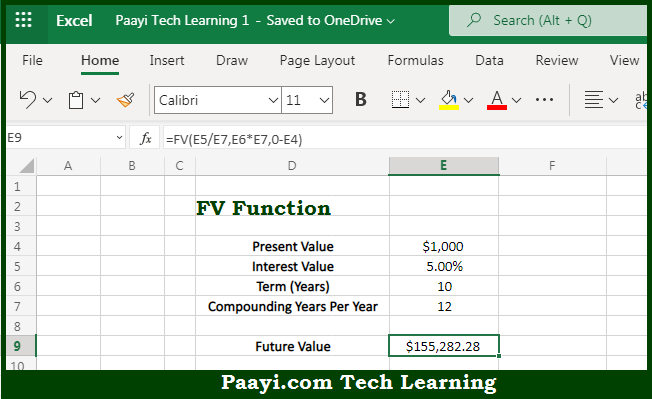# Learn How to Use Microsoft Excel FV Function

Written by | 0 Comments | 625 Views

In this article, you will learn how to use the Microsoft Excel FV function and its prime function in Microsoft Excel. You will also get to know the Microsoft Excel FV function return value and syntax with the help of some examples.

Microsoft Excel FV Function

The main purpose of the Microsoft Excel FV function is to get the future value of an investment. That implies, with the help of the FV function you can able to return the future value of an investment. You can use the FV function to get the future value of an investment assuming periodic, constant payments with a constant interest rate. So, with the help of the FV function, you can able to get the future value of an investment.

Return Value of FV Function

The return value will be the future investment value.

Syntax of FV Function

=FV(rate, nper, pmt, [pv], [type])

Where the arguments:

• rate: This is the interest rate period.
• nper: This is the total number of payment periods.
• pmt: This is the payment made each period. Must be entered as a negative number.
• pv: This is the present value of future payments. In case omitted, assumed to be zero. Must be entered as a negative number.
• type: This is when payments are due. 0 = end of period, 1 = beginning of period. Default is 0 (optional).

How to Use Microsoft Excel FV Function?So we know that Microsoft Excel FV function you can able to get the future value of an investment. That implies, with the help of the FV function you can able to return the future value of an investment. You can use the FV function to get the future value of an investment assuming periodic, constant payments with a constant interest rate. So, with the help of the FV function, you can able to get the future value of an investment.